﻿ MotionDesigner Reference & User Interface > Motion Laws / Cam-Laws for Segments > Constant-Velocity

# Motion-Law: Constant-Velocity

### Constant-Velocity Cam-Law, Motion-Law

This is a Polynomial motion type

Motion-Description

 The Velocity is Constant for the period of the segment-width.

Motion-Values

You CAN control the:

 Start-Position The Start-Position usually flows from the End-Position of the Start-Velocity The Start-Velocity usually flows from the End-Velocity of the Previous-Segment

You CANNOT control the:

 End-Position The End-Position is a calculated from the Start-Position, Start-Velocity, and Segment-Width End-Velocity The End-Velocity is equal to its Start-Velocity End-Acceleration End-Jerk

### Example

Velocity Continuity

 1.Use the Motion-Law Selector to change a motion-law of a segment to a Constant-Velocity. 2.Click above or below the segment in the motion-graphs. The Constant-Velocity segment is now the Selected-Segment. 3.Open the Blend-Point Editor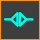1.Set the Match Control Button to Flow / Match - see image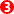The Velocity at the Start of the Selected-Segment is now forced to be equal to the Velocity at the End of the Previous-Segment. The Start Velocity becomes read-only. 2.Edit the End VelocitySee that the Start-Velocity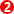is always equal to the End-Velocity.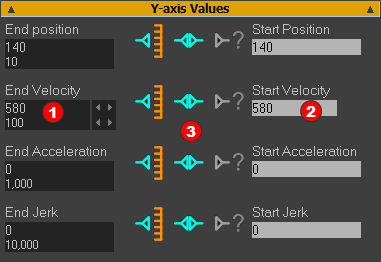Blend-Point Editor: Velocity Equal at Blend-Point.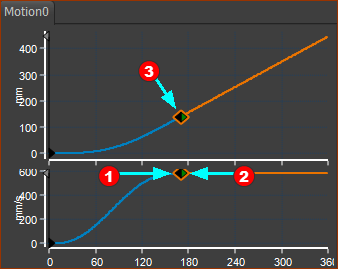Smooth Flow of Velocity at Blend-Point Equal Gradients of Position Graph at Blend-Point

### Example continued...

VELOCITY DIS-CONTINUITY

Definition of dis-continuity: the motion-value of the motion-derivative changes instantly, with a step. A Dis-continuity is also called a Step-Change.

A discontinuity in Velocity is not recommended, of course.

A discontinuity in Velocity is also infinite acceleration - mechanically impossible.

A discontinuity puts a kink in the Position graph.

An example of a Velocity Dis-Continuity.

 1.Use the Motion-Law Selector to change a motion-law of a segment to a Constant-Velocity. 2.Click above or below the segment in the motion-graphs. The Constant-Velocity segment is now the Selected-Segment. 3.Open the Blend-Point Editor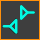4.Set the Match Control-Button to Do Not Match5.Enter a Start-Velocityvalue that is not equal to the End-Velocityvalue.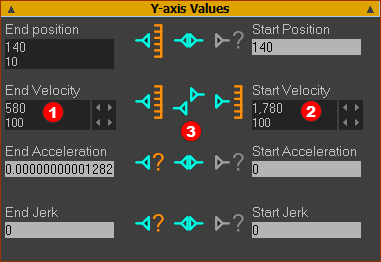Blend-Point Editor: Velocities at Blend-Point are not Equal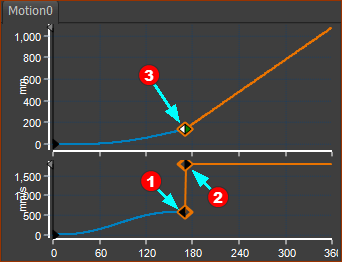Step in Velocity Graph, & Kink(corner) in Position Graph. Step in gradient at Blend-Point in Position-Graph.